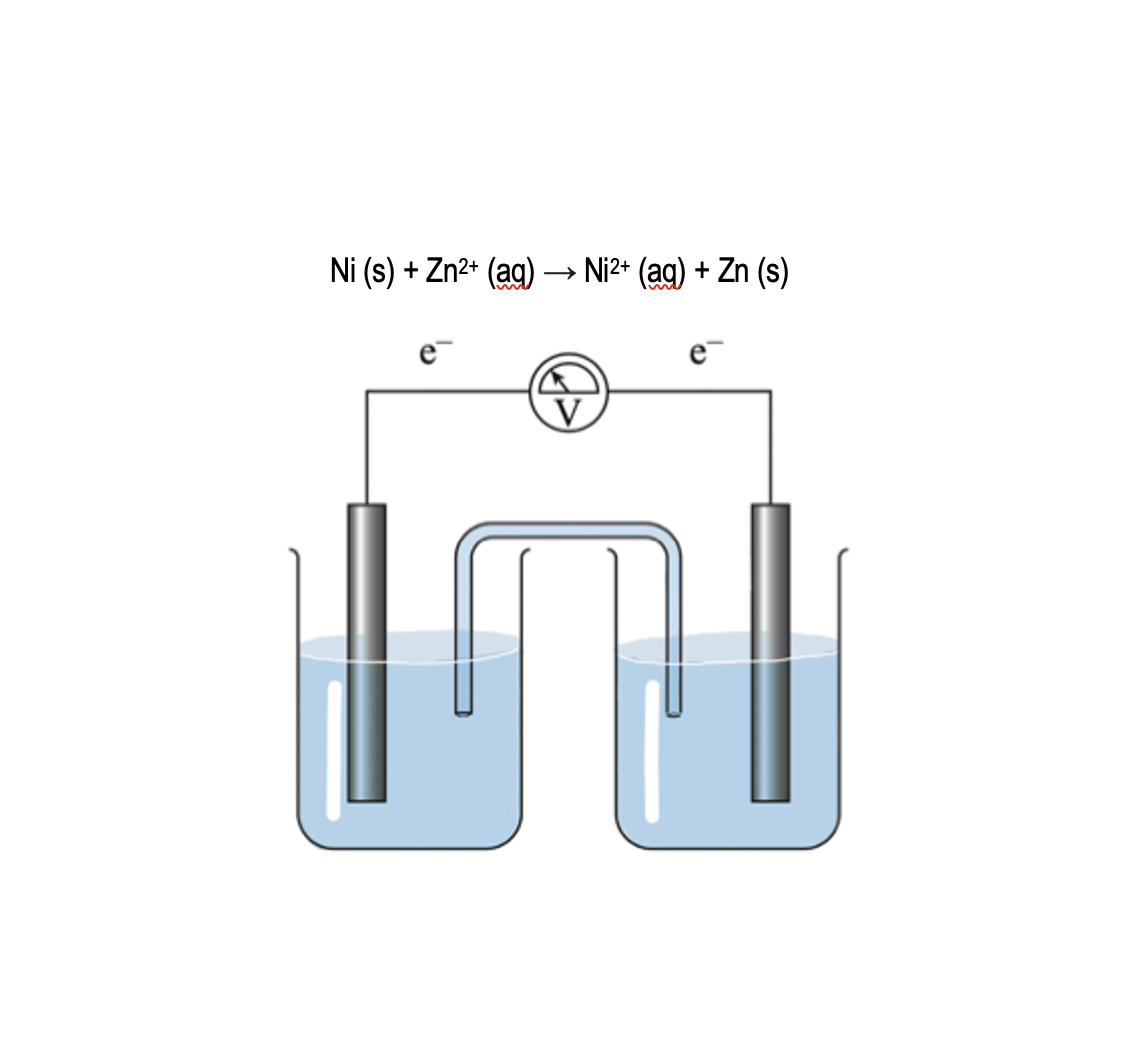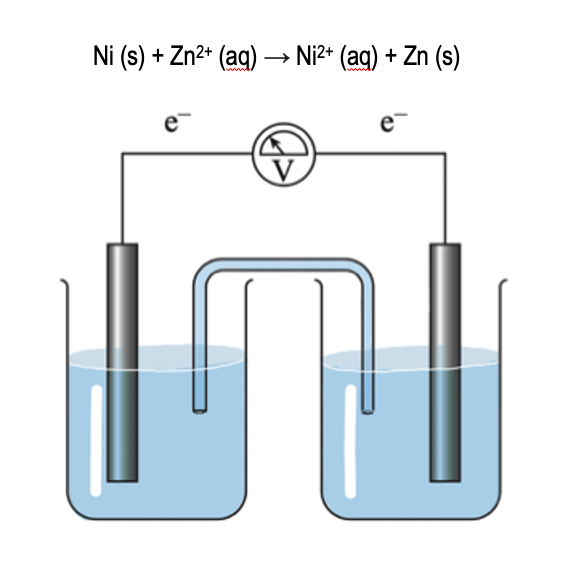Clutch Prep is now a part of Pearson
Ch.18 - ElectrochemistryWorksheetSee all chapters

# Galvanic Cell

See all sections
Sections
Standard Reduction Potentials
Intro to Electrochemical Cells
Galvanic Cell
Electrolytic Cell
Cell Potential: Standard
Cell Potential: The Nernst Equation
Cell Potential and Gibbs Free Energy
Cell Potential and Equilibrium
Cell Potential: G and K
Cell Notation
Electroplating

A Galvanic Cell represents a spontaneous electrochemical cell

###### Examining a Galvanic Cell

Concept #1: A Galvanic Cell uses a spontaneous redox reaction in order to produce electricity.

Example #1: The purpose of a galvanic cell is to:

a) Purify solids

b) Allow for only oxidation

c) Generate electricity

d) To consume electricity

Concept #2: A typical galvanic cell has major components that harness the power of a spontaneous redox reaction.

Example #2: Which of the following statements is true for a salt bridge dealing with the following redox reaction:

Mg (s) + Cd2+ (aq) → Mg2+ (aq) + Cd (s)

a) Its bromide ions will flow to the magnesium half-cell.

b) Its bromide ions will flow to the cadmium half-cell.

c) Contains neutral atoms that interact with the ions in both half-cell compartments.

d) Its sodium ions will flow to the magnesium half-cell.

Concept #3: The anode decreases in mass and the cathode increases in mass over time.

Example #3: How many electrons would be transferred between a sodium and gallium electrode from the following?

Ga3+ (aq) + 3 e → Ga (s)

Na (s) ­ → Na+ (aq) + e –

Concept #4: Galvanic Cells represent spontaneous electrochemical cells.

Example #4: A reduction reaction with an equilibrium constant of 4.8 x 102

a) Is nonspontaneous and has a positive Eºcell

b) Has a negative ∆Gº and produces electricity

c) Has discharged all electricity and is a dead battery

d) Is spontaneous and has a negative Eºcell

Which of the following is false about a Voltaic cell?

For the following redox reaction label: the anode, cathode, half-reactions occurring at each half-cell, direction of electron flow, and direction of neutral ions flow.